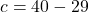## Use the table to write and solve an equation to find the number of cars c that a salesperson needs to sell in the fourth month so that

Question

Use the table to write and solve an equation to find the number of
cars c that a salesperson needs to sell in the fourth month so that
the salesperson’s mean number of cars sold per month is 10.
Month 1 2 3 4
Cars Sold 8 9 12 c

in progress 0
2 weeks 2021-08-30T19:18:53+00:00 1 Answers 0 views 0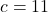Step-by-step explanation:

Given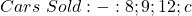Required

What value of c makes the mean = 10

Mean is calculated as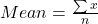In this case;

Mean = 10 and n = 4;

So, we have: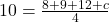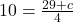Multiply both sides by 4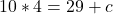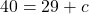Solve for c